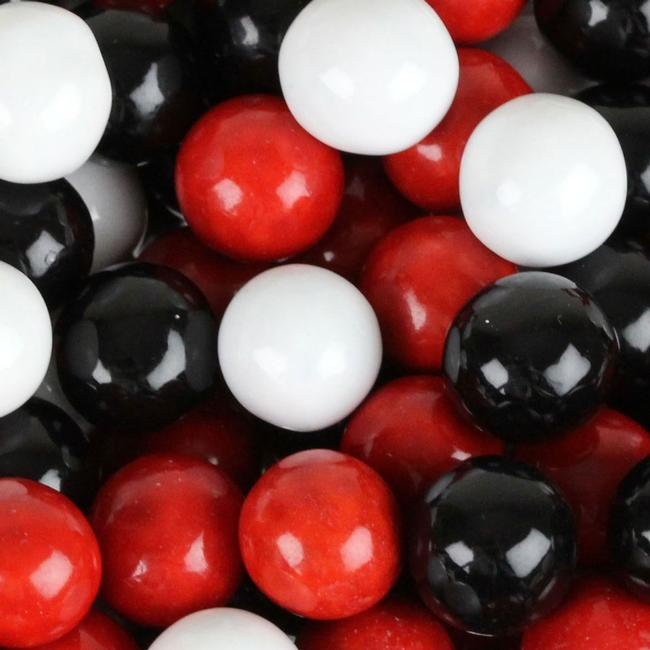# A Difficult Choice!

Probability Level 2A box contains 4 balls. The color of each of the balls is one of the three: White, Black or Red. However, you don't know how many balls of each color are there. It might even be the case that all the balls are of the same color. You then draw two balls randomly from the box. The probability that both the drawn balls are red can be written as $\dfrac{p}{q}$, where $p$ and $q$ are coprime positive integers. What is the value of $p+q$?

×